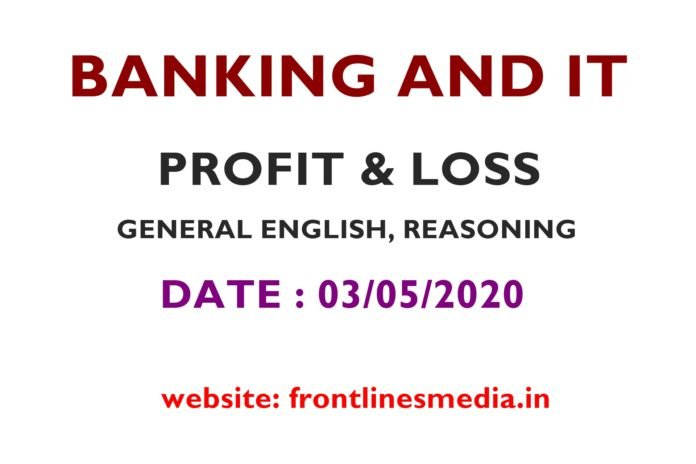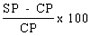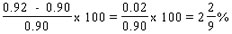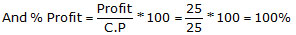# BANKING & IT JOBS TEST: PROFIT AND LOSS, DATE: 03/05/2020

0
1936Answer all the questions to get the result

# BANKING & IT

We at Frontlines Media are giving problems, daily questions, and a test on  Sunday. By, which one can get a clear idea and solving capacity of every topic. This is our third test after the Number system and Percentages. This test will be on Profit and Loss. All the best.

#### BANKING & IT

Question 1 of 25.

1. 3750
2. 4000
3. 2250
4. 4750

### 3750

CP = (Difference in SP) ÷ (% Difference in profit) = 600/(5-(-11)) × 100 = (600/16)×100 = Rs3750

Question 2 of 25.

1. 14%
2. 10%
3. 18%
4. 12.5%

### 10%

Let CP= Rs. 100, therefore, MP= Rs. 125, Discount% = 12%
SP = 88% of MP = 88% of 125 = Rs. 110. Profit = 110 – 100 = Rs. 10
Profit = 10%.

Question 3 of 25.

1. 6
2. 5.80
3. 6.25
4. 7.70

### 7.70

Rs. 1.4 is the increase in absolute, 20% is the increase in percentage. Thus those two can be equated. Hence it can be concluded that 1.4 is 20% of the price i.e. the CP of one orange is 1.4/0.20 = Rs. 7. The SP per orange in the first case is 7 × 1.1 = Rs. 7.70.

Question 4 of 25.

1. 10%
2. 25%
3. 42%
4. 20%

### 10%

Such question can be better answered by thinking in the reverse order. Let the CP = 100, there is a loss of 12% in the final case i.e. SP = 100 – 12 = Rs 88. Now this Rs. 88 is the four-fifth of the selling price as per the question. The original selling price will be 88 × 5/4 = Rs. 110. CP =100. There is a profit of Rs. 10, which is nothing but 10%, as the CP is taken to be Rs. 100

Question 5 of 25.

### A trader mixes 26 kg of rice at Rs. 20 per kg with 30 kg of rice of other variety at Rs. 36 per kg and sells the mixture at Rs. 30 per kg. His profit percent is:

1. No profit, no loss
2. 5%
3. 8%
4. 10%

### 5%

c.P. of 56 kg rice = Rs. (26 x 20 + 30 x 36) = Rs. (520 + 1080) = Rs. 1600.

S.P. of 56 kg rice = Rs. (56 x 30) = Rs. 1680.

Gain =(80/1600*100) % = 5%

Question 6 of 25.

### If books bought at prices ranging from Rs. 200 to Rs. 350 are sold at prices ranging from Rs. 300 to Rs. 425, what is the greatest possible profit that might be made in selling eight books ?

1. 600
2. 1200
3. 1800
4. none of the above

### 1800

Least Cost Price = Rs. (200 * 8) = Rs. 1600.

Greatest Selling Price = Rs. (425 * 8) = Rs. 3400.

Required profit = Rs. (3400 - 1600) = Rs. 1800.

Question 7 of 25.

1. 10000
2. 12000
3. 13000
4. 14000

### 10000

Cost Price = Rs.[100125×8750]Rs.100125×8750 = Rs. 7000. Let the labelled price be Rs. x.

Then, 70100*x=700070100*x=7000 x=Rs.(7000*10070)⇒x=Rs.7000*10070

= Rs.10000

Question 8 of 25.

1. 23
2. 24
3. 25
4. none of them

### 24

Cost Price of 5 kg = Rs.(14*2 + x*3) = (28 + 3x).

Sell price of 5 kg = Rs. (22x5) = Rs. 110.

[{110 - (28 + 3x)}/(28 + 3x) ]* 100 =10

[82-3x/28 + 3x]= 1 / 10

820 - 30x = 28 +3x

33x = 792

x = 24

Question 9 of 25.

1. 240
2. 360
3. 420
4. 600

### 420

Initially                 CP           profit            SP                MP

100           x               (100+x)         133.33

After Change     100           2x             (100+x)

Now,   Since (100+x)  - 100 = 2x

7676 x= 20%

CP            Profit              SP             MP

100             20               120           133.33

So,                  300             60               360             400

Again             300            120              420

So the increased selling price = Rs. 420

Question 10 of 25.

1. 404.80
2. 536.80
3. 440
4. 160

### 404.80

Let C1 be the cost price of the first article and C2 be the cost price of the second article.
Let the first article be sold at a profit of 22%, while the second one be sold at a loss of 8%.

We know, C1 + C2 = 600.
The first article was sold at a profit of 22%. Therefore, the selling price of the first article = C1 + (22/100)C1 = 1.22C1
The second article was sold at a loss of 8%. Therefore, the selling price of the second article = C2 - (8/100)C2 = 0.92C2.

The total selling price of the first and second article = 1.22C1 + 0.92C2.

As the merchant did not make any profit or loss in the entire transaction, his combined selling price of article 1 and 2 is the same as the cost price of article 1 and 2.

Therefore, 1.22C1 + 0.92C2 = C1+C2 = 600
As C1 + C2 = 600, C2 = 600 - C1. Substituting this in 1.22C1 + 0.92C2 = 600, we get
1.22C1 + 0.92(600 - C1) = 600
or 1.22C1 - 0.92C1 = 600 - 0.92*600
or 0.3C1 = 0.08*600 = 48
or C1 = 48/(0.3) = 160.
If C1 = 160, then C2 = 600 - 160 = 440.
The item that is sold at loss is article 2. The selling price of article 2 = 0.92*C2 = 0.92*440 = 404.80.

Question 11 of 25.

### A person bought an article and sold it at a loss of 10 % if he had bought it for 20 % less and sold it for Rs. 55 more he would have had a profit of 40 %. The C.P of the article is:

1. 200
2. 225
3. 250
4. None of the above

### 250

C.P = Rs. X

S.P = Rs. (90/100 × X) = Rs. (9/10 X)

C.P = Rs. (80/100 × X) = Rs. (4X/5) Gain = 40%

New S.P = Rs. (140/100 × 4X/5)

= Rs. (28/25 X) 28/25 X – 9/10 X = 55 or X = 250

Question 12 of 25.

### At what price must kantilal sell a mixture of 80 kg sugar at Rs. 6.75 per kg with 120 kg at Rs. 8 per kg to gain 20%?

1. Rs. 7.50 per kg
2. Rs. 8.20 per kg
3. Rs. 8.85 per kg
4. Rs. 9 per kg

### Rs. 9 per kg

C.P of 200 kg of sugar = Rs. (80 × 6.75 + 120 × 8) = Rs. 1500

C.P of 1 kg = Rs. (1500/200) = Rs. 7.50

Gain required = 20%

S.P of 1 kg = (120% of Rs. 7.50)

= Rs. (120/100 × 7.50) = Rs. 9 per kg

Question 13 of 25.

### Kajal bought paper sheets for Rs. 7200 and spent Rs. 200 on transport. Paying Rs 600 she had 330 boxes made. Which he said at Rs. 28 each. What is her profit Percentage?1. 15.5
2. 40
3. 60
4. None of the above

### 15.5

330 boxes = Rs. (7200 + 200 600) = Rs. 8000

330 boxes = Rs. (330 × 28) = Rs. 9240

Gain% = (1240/8000 × 100)% = 15.5%

Question 14 of 25.

1. 14.40
2. 16
3. 16.80
4. 19.20

### 16

6 dozen apples = Rs. (12 × 4 + 16 × 2) = Rs. 80

Gain = 20%

S.P = Rs. (120/100 × 80) = Rs. 96

Per dozen = Rs. (96/6) = Rs. 16

Question 15 of 25.

1. 1/3
2. 1/8
3. 2/8
4. 1/16

### 1/8

It’s a division series. Every number is half of the previous number. The number is divided by 2 successively to get the next result. 4/2 = 2. 2/2 = 1. 1/2 = ½. (1/2)/2 = ¼. (1/4)/2 = 1/8 and so on.

Question 16 of 25.

1. index
2. glossary
3. Chapter
4. Book

### Book.

Rest are all parts of a book.

Question 17 of 25.

### Safe : Secure :: Protect :

1. lock
2. guard
3. sure
4. conserve
Question 18 of 25.

### The chairman is ill and we will have to ........ the meeting for few days

1. put on
2. put of
3. put away
4. put off
Question 19 of 25.

### The cat and dog have a ............. enemy in the rat

1. same
2. common
3. mutual
4. similar
Question 20 of 25.

### He is very good ...... making stories.

1. in
3. at
4. for
Question 21 of 25.

### One year payment to the servant is Rs. 200 plus one shirt. The servant leaves after 9 months and recieves Rs. 120 and a shirt. What is the price of the shirt?

1. 80
2. 100
3. 120
4. None of the above

### 120

The servant worked for 9 months instead of 12 months, he should receive 9/12 of his annual payment

Let the price of 1 shirt be Rs.S.

i.e.,.

However, the question states that the servant receive Rs. 120 + S where S is the price of the shirt.

By equating the two equations we get= 120 + S.

Therefore Price of the shirt S = Rs. 120.

Question 22 of 25.

1. 2% loss
2. 2.22% gain
3. 2% gain
4. None of these

### 2.22% gain

The trader professes to sell his goods at a loss of 8%.
Therefore, Selling Price = (100 - 8)% of Cost Price
or SP = 0.92CP

But, when he uses weights that measure only 900 grams while he claims to measure 1 kg.
Hence, CP of 900gms = 0.90 * Original CP

So, he is selling goods worth 0.90CP at 0.92CP
Therefore, he makes a profit of 0.02 CP on his cost of 0.9 CP

Profit % =i.e.,or 2.22%

Question 23 of 25.

1. 28.57%
2. 40%
3. 66.66%
4. 58.33%

### 66.66%

If the merchant offers a discount of 40% on the marked price, then the goods are sold at 60% of the marked price.

The question further states that when the discount offered is 40%, the merchant sells at cost price.

Therefore, selling @ 40% discount = 60% of marked price (M) = cost price (C)ori.e., a mark up 66.66%

Question 24 of 25.

1. 25%
2. 50%
3. 100%
4. 66.7%

### 100%

Let the selling price per article be = Re. 1
Therefore, selling price of 50 articles = Rs.50

Profit on sale of 50 articles = selling price of 25 articles = Rs.25.
S.P = 50. Profit = 25. Therefore, CP = 50 - 25 = 25..

Question 25 of 25.

### I came here to discuss ........... cricket with him

2. on
3. None of the above

#### Next question 1 of 25

All 25 questions completed!

# Want more stuff like this?

Dont worry, we dont spam